EnchantedLearning.com is a user-supported site.
As a bonus, site members have access to a banner-ad-free version of the site, with print-friendly pages.

 You might also like: U: Illustrated Math Dictionary - Enchanted Learning.com L: Illustrated Math Dictionary - Enchanted Learning.com Q: Illustrated Math Dictionary - Enchanted Learning.com Z: Illustrated Math Dictionary - Enchanted Learning.com R: Illustrated Math Dictionary - Enchanted Learning.com Today's featured page: Identifying Fractions

 +, - EnchantedLearning.comMath Dictionary x, ÷
 A B C D E F G H I J K L M N O P Q R S T U V W X Y ZM is for ...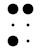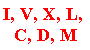M

M equals 1000 in Roman numerals.
 5 4 6 6 5 4 4 6 5

magic square

In a magic square, the rows, columns, and diagonals all add up to the same number.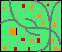map

A map (also called a cartograph) shows the features of an area. You can find your way around by using a map.
 mathematicianA mathematician is a person who studies mathematics.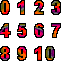mathematicsMathematics is the study of numbers, shapes, patterns, and logical reasoning.mazeGetting through the passages of a maze is tricky. mean, arithmeticThe arithmetic mean of a set of numbers (also called the average) is equal to the sum of the numbers divided by the number of numbers. For example, for the data set {1, 2, 3, 6}, the mean = (1+2+3+6)/4 = 12/4 = 3.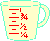measurementMeasurement is the act of determining size, weight, volume, amount, capacity, or degree. For example, a measuring cup is useful for determining the amounts of ingredients when cooking. {1, 2, 5, 8, 10}medianThe median of a set of numbers is the middle number (when the numbers are in order). When the number of numbers is odd, the median is the middle number; when the number of numbers is even, the median is the average of the two middle numbers. For example, the median of the set {1,2,5,8,10} is 5. The median of the set {1,2,5,6,9,10} is 5.5.medianThe median of a triangle is a line segment from a vertex to the midpoint of the opposite side. The median of a trapezoid is a line segment that connects the midpoints of the non-parallel sides. mental mathMental math is math that is done in your head, without writing or using a calculator or other device.
 milliMilli is a prefix that means one-thousandth. For example, a millimeter is one thousandth of a meter. millionA million is 1,000,000 or one thousand thousand or 106.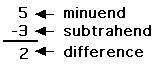minuendThe minuend is the number from which another number is subtracted in a subtraction problem. -minus signThe mathematical symbol - means "minus." Four minus three is written, 4 - 3.
 minuteA minute is a measure of angle that is equal to 1/60th of a degree. minuteA minute is a measure of time that is 1/60th of an hour. A minute is composed of 60 seconds.minute handA minute hand on a clock tells you how many minutes past the hour it is. 1 2/3mixed numberA mixed number is a number that is written as a whole number plus a fraction, like 1 2/3.Möbius stripA Möbius strip is a piece of paper with only one side! modeThe mode of a group of numbers is the number that occurs the most in that set. For example, in the set {1, 2, 3, 3, 3, 8}, the mode is 3. modular arithmeticModular arithmetic is arithmetic done with a limited set of numbers. For example, clock arithmetic is an example of modular arithmetic; when adding hours, 11+2=1 (and not 13).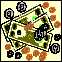moneyMoney is used as a basis for trade. Most money is decimal (based on the number 10). Only a few countries have money with another base (like Madagascar and Mauritania, which have base 5 money). monomialA monomial is a polynomial expression with only one term. For example, 3xy is a monomial; the number 6 is also a monomial.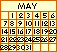monthThere are 12 months in a year: January, February, March, April, May, June, July, August, September, October, November, and December. Müller, Johann Johann Müller, also known as Johann Regiomontanus (1436-1476) was a German astronomer and mathematician. He studied trigonometry, translating Ptolemy's Almagest, from the original Greek. Ironically, his translation helped overthrow the Ptolemaic view of the universe (in which the Earth was thought to be at the center of the universe). He also did work on plane and spherical trigonometry. Muller also observed the motion of the moon, planets, and comets. A 108 km diameter lunar crater, called Regiomontanus (latitude -28.3 degrees, longitude 1.0 degrees), was named for Muller. Multiples of 2:2,4,6,8,10,12,...multipleA multiple of a number is the product of the original number and another number. For example, the multiples of the 2 are 2, 4, 6, 8, 10, 12, 14, 16, and so on.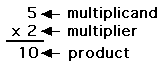multiplicandThe multiplicand is the number that is multiplied by the multiplier in a multiplication problem. 1 x 2 = 22 x 3 = 6multiplicationMultiplication is a mathematical operation used to compute areas and volumes, and also in many other calculations. 1multiplicative identityThe multiplicative identity (also called the identity for multiplication) is one, because a x 1 = 1 x a = a. For example, 2x1=1x2=2. multiplicative inverseThe multiplicative inverse of a is 1/a (where a is not equal to zero), since for every non-zero number a, a x (1/a) = 1.multiplierThe multiplier is the number by which the multiplicand is multiplied.

 +, - EnchantedLearning.comMath Dictionary x, ÷
 A B C D E F G H I J K L M N O P Q R S T U V W X Y Z
 Counting Addition Subtraction Multiplication Division
 Number Line Fractions Decimals Graphs Measurement Rounding

Click on an underlined word for more information on that subject. If the math term you are looking for is not in the dictionary, please e-mail us.

Enchanted Learning®
Over 35,000 Web Pages
Sample Pages for Prospective Subscribers, or click below

 Overview of Site What's New Enchanted Learning Home Monthly Activity Calendar Books to Print Site Index K-3 Crafts K-3 Themes Little ExplorersPicture dictionary PreK/K Activities Rebus Rhymes Stories Writing Cloze Activities Essay Topics Newspaper Writing Activities Parts of Speech Fiction The Test of Time iPhone app TapQuiz Maps - free iPhone Geography Game Biology Animal Printouts Biology Label Printouts Biomes Birds Butterflies Dinosaurs Food Chain Human Anatomy Mammals Plants Rainforests Sharks Whales Physical Sciences: K-12 Astronomy The Earth Geology Hurricanes Landforms Oceans Tsunami Volcano Languages Dutch French German Italian Japanese (Romaji) Portuguese Spanish Swedish Geography/History Explorers Flags Geography Inventors US History Other Topics Art and Artists Calendars College Finder Crafts Graphic Organizers Label Me! Printouts Math Music Word Wheels

## Enchanted Learning Search

 Search the Enchanted Learning website for: